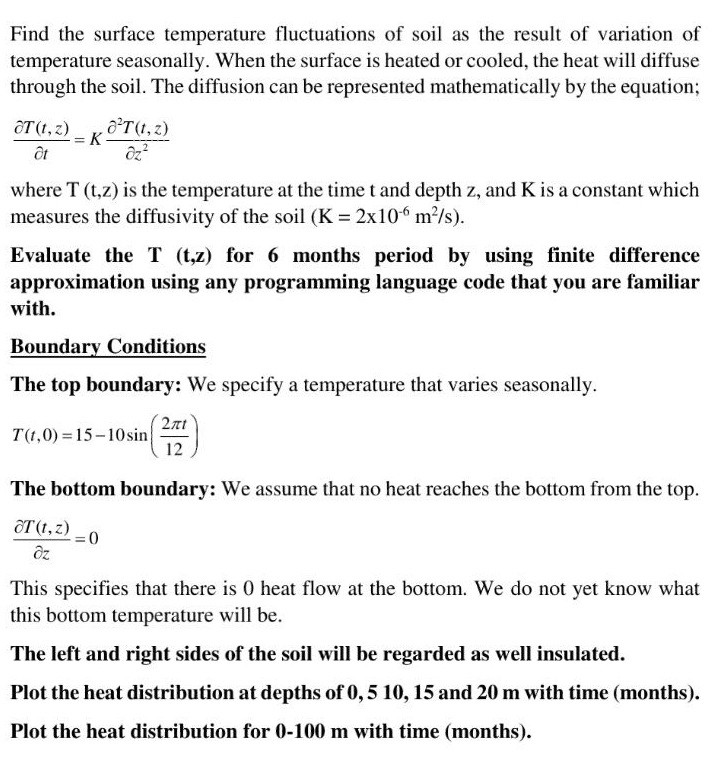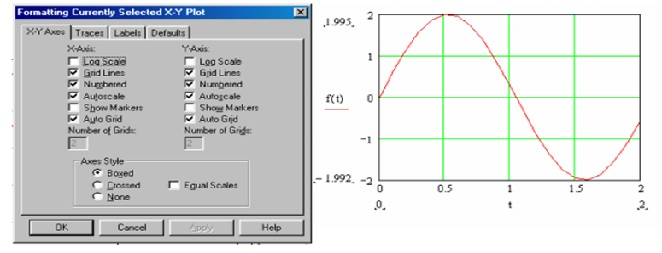# Question & Answer: Hello, can you solve the graphical solution of the question using the mathcad program? Thank you……Hello, can you solve the graphical solution of the question using the mathcad program? Thank you.

Don't use plagiarized sources. Get Your Custom Essay on
Question & Answer: Hello, can you solve the graphical solution of the question using the mathcad program? Thank you……
GET AN ESSAY WRITTEN FOR YOU FROM AS LOW AS \$13/PAGE

Find the surface temperature fluctuations of soil as the result of variation of temperature seasonally. When the surface is heated or cooled, the heat will diffuse through the soil. The diffusion can be represented mathematically by the equation: partial differential T(t, z)/partial differential t = K partial differential^2 T(t, z)/partial differential z^2 where T (t, z) is the temperature at the time t and depth z, and K is a constant which measures the diffusivity of the soil (K = 2 times 10^-6 m^2/s). Evaluate the T (t, z) for 6 months period by using finite difference approximation using any programming language code that you are familiar with. Boundary Conditions The top boundary: We specify a temperature that varies seasonally. T(t, 0) = 15 – 10 sin (2 pi t/12) The bottom boundary: We assume that no heat reaches the bottom from the top. partial differential T(t, z)/partial differential z = 0 This specifies that there is 0 heat flow at the bottom. We do not yet know what this bottom temperature will be. The left and right sides of the soil will be regarded as well insulated. Plot the heat distribution at depths of 0, 5 10, 15 and 20 m with time (months). Plot the heat distribution for 0-100 m with time (months).

How to solve graphs using MATHCAD

Let’s assume to plot v = 2 cos(ωt)

You wish to plot v vertically against time t horizontally.

1. Initialize the starting and finishing values for time of the plot and the intervals at which you wish to plot your points. Like t=1, 2, 3…… and so on. Here the first point is 1 and last point can be any point you wish.
1. Instead of using the symbol ‘v’ we have to recognize that v is a function of time ‘t’ and so we write f(t) = 2 cos(ωt).

First we must write down the value of the constants such as ω. Ex: ω=2 / ω=5/ ω=8 any value.

1. Place the curser at a suitable point and type ‘f(t) =’

When you press enter with ‘=’ the table of values should appear for all f(t).

1. Then we need to place the cursor where we want our graph to appear. On the graph tool bar click x – y plot command and the plot frame will appear. On the horizontal axis enter ‘t’ and on the vertical axis enter ‘f(t)’

So as of now values stands are: t=1, 2, 3, 4, 5; ω=5; f(t)=2.cos(ω.t) <All values are taken be me for example>

1. Next click on the plot to highlight it and then on the menu bar click on format graph then x – y plot and obtain the following window. Click on grid lines or any other thing that you want such as labels and plot colors to produce your plot in its final form.<All values are taken be me for example>